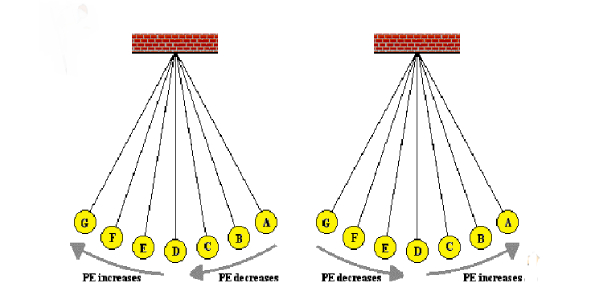# Ocr P4 21st Century Quiz. Potential Energy, Kinetic Kinergy

10 Questions | Attempts: 2024
ShareSettingsThis quiz aims to test basic knowledge of the Physics topic covering Gravitational Potential Energy, Kinetic Energy and Work Done by a Force on an object. It also explores conservation of energy and the consequences of friction and air resistance.

• 1.
What name is given to the energy of a moving object? E.g. A Car.
• A.

Heat

• B.

Gravitational Potential Energy

• C.

Electrical Potential Energy

• D.

Atomic Energy

• E.

Kinetic Energy

• 2.
A stone is dropped from the top of a cliff. How does the energy of the stone change as it falls from the top of the cliff to the ground below?
• A.

Kinetic Energy of the stone transforms to gravitational potential energy.

• B.

Kinetic energy of the stone is dissipated as heat.

• C.

Potential energy of the stone transforms to kinetic energy.

• D.

There is no change is potential energy.

• E.

There is no change in kinetic energy.

• 3.
If the mass of an object is multipled by the Graviational Field Strength (g for earth), what does this give?
• A.

The objects height.

• B.

The objects width.

• C.

The objects weight.

• D.

The total energy of the object.

• E.

The density of the object.

• 4.
If the kinetic energy of an object is doubled, how many times will its velocity increase by?
• A.

10 times

• B.

5 times

• C.

4 times

• D.

None at all

• E.

2 times

• 5.
You are rolling a football along the ground. You get tired and you stop with the ball. What happened to the gravitational potential energy of the ball?
• A.

Increased.

• B.

Decreased.

• C.

Remained Zero.

• D.

None of the above.

• E.

Energy doesn't exist. Humans invented the idea to explain the unexplainable.

• 6.
A man is walking at 1 metre per second (m/s). He has a mass of 100 kilogram (Kg). How much kinetic energy does he have?
• A.

He doesn't have any kinetic energy.

• B.

6 Mega (6,000,000) Electron Volts.

• C.

5 Joules.

• D.

50 Joules.

• E.

100 Joules.

• 7.
What is the equation for the Work Done by a force on an object?
• A.

Gravitational Field Strength multipied by the mass of the object.

• B.

Force applied to an object multiplied by the distance the object moves. ( In the direction of the force).

• C.

The work done on an object does not depend on the force applied.

• D.

Kinetic energy of the object multiplied by the Gravitational Potential Energy of an object.

• E.

Force applied to an object divided by the distance the object moves ( In the direction of the force).

• 8.
An object is 5 metres high off the ground on earth. The object is then placed 5 metres high on the moon's surface. Assuming that the gravitational field strength on the moon is only half that of the earth, how does the gravitational potential energy of the object change on the moon?
• A.

It has doubled.

• B.

It has tripled.

• C.

There is no gravity in the whole of space, everything just floats around randomely.

• D.

It is halved

• E.

There is no change

• 9.
Your science teacher slides a book across the work bench or table. The book eventually stops after sliding. Is energy conserved in this situation?
• A.

All of the answers are true.

• B.

None of the answers can be true.

• C.

Yes. Energy is conserved. The Work done by the science teacher equals the change in energy of the book.

• D.

No. Friction opposes the motion of the book and the energy is dissipated as heat to the surroundings.

• E.

There is no such thing as conservation of energy.

• 10.
An object moves a distance of 10 metres due to a force pushing it to the right. How much work is done on the object in the direction, which is perpendicular to the direction off travel?
• A.

20 Joules

• B.

10 Joules.

• C.

No work is done.

• D.

Same amount in all directions.

• E.

300 Joules.

## Related TopicsBack to top
×

Wait!
Here's an interesting quiz for you.